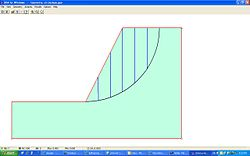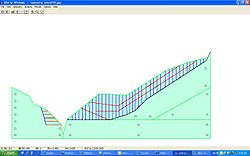# Discontinuous Deformation Analysis

Discontinuous Deformation Analysis

Discontinuous Deformation Analysis (DDA) is a type of discrete element method originally proposed by Shi in 1988. DDA is somewhat similar to the finite element method for solving stress-displacement problems, but accounts for the interaction of independent particles (blocks) along discontinuities in fractured and jointed rock masses. DDA is typically formulated as a work-energy method, and can be derived using the principle of Minimum Potential Energy (e.g., Shi) or by using Hamilton's principle. Once the equations of motion are discretized, a step-wise linear time marching scheme in the Newmark family is used for the solution of the equations of motion. The relation between adjacent blocks is governed by equations of contact interpenetration and accounts for friction. DDA adopts a stepwise approach to solve for the large displacements that accompany discontinuous movements between blocks. The blocks are said to be "simply deformable". Since the method accounts for the inertial forces of the blocks' mass, it can be used to solve the full dynamic problem of block motion.

## DDA vs DEM

Although DDA and DEM are similar in the sense that they both simulate the behavior of interacting discrete bodies, they are quite different theoretically. While DDA is a displacement method, DEM is a force method. While DDA uses displacement as variables in an implicit formulation with opening-closing iterations within each time step to achieve equilibrium of the blocks under constrains of the contact, DEM employs an explicit, time marching scheme to solve the equations of motion directly (Cundall and Hart, 1989). The system of equation in DDA is derived from minimizing the total potential energy of the system being analyzed. This guarantee that equilibrium is satisfied at all times and that energy consumption is natural since it is due to frictional forces. In DEM, unbalanced forces drive the solution process, and damping is used to dissipate energy. If a quasi-static solution is desired in which the intermediate steps are not of interest, the type of damping and the type of relaxation scheme can be selected in DEM to obtain the most efficient solution method (Cundall, 1987). The application of damping in DEM for quasi-static problem is somewhat analogues to the setting to zero of the initial velocities of the block in the static analysis of DDA. In dynamic problem, however, the amount and type of damping in DEM, which are very difficult to qualify experimentally, has to be selected very carefully to as not to damp out real vibrations. On the other hand, the energy consumption in DDA is due to the frictional resistance at contact. By passing the velocities of the blocks at the end of a time step to the next time step, DDA gives real dynamic solution with correct energy consumption (Shi, 1988). By using an energy approach, DDA does not require an artificial damping term to dissipate energy as in DEM, and can easily incorporate other mechanisms for energy loss.

## Strengths and Limitations of the DDA method

DDA has several strengths recommending it for use in slope stability problems in jointed rock masses, which are balanced by serious limitations be accounted when DDA is used for larger scale, faster moving problems.

### Strengths

• Very good for problems with small characteristic as time marching scheme provides necessary numerical damping to control resonance interactions within and between particles.
• Step-wise linear implicit time marching allows so-called quasi-static solutions, where step-wise velocities are never used. Quasi-static analysis is useful for examining slow, or creeping failures.

### Limitations

• The most serious limitation of the DDA method is the reduction of numerical damping which occurs as the characteristic length of a problem grows. The numerically, damping is a function of$\sqrt{k/M}$. Typically,

the stiffness k doesn't vary over 1 or 2 orders of magnitude, while the mass M is a function of the cube of the characteristic length.

## Modification and improvement of the DDA method

Various modifications to the original DDA formulation have been reported in the rock mechanics literature. In the original DDA formulation a first order polynomial displacement function was assumed, so the stresses and strains within a block in the model were constant. This approximation precludes the application of this algorithm to problems with significant stress variations within the block. However, in cases where the displacement inside the block is high and cannot be ignored, the blocks can be divided by mesh. An example of this approach is the research by Chang et al. and also Jing who resolved this problem by adding finite element meshes in the two-dimensional domain of the blocks so that stress variations within the blocks can be allowed.

Higher order DDA method for two-dimensional problems has been developed in both theory and computer codes by researchers like Koo and Chern, Ma et al. and Hsiung. Additionally, Roozbeh Grayeli and Ali Mortazavi implemented finite element mesh based on six node triangular element into the original DDA blocks in order to account for stress variations within the blocks. The DDA contact model which was originally based on penalty method was improved by adopting the Lagrange type approach reported by Lin et al.

Since a blocky system is a high non-linear system due to non-linearity within blocks and between blocks, Chang et al. implemented a material non-linearity model to DDA using strain hardening curves. Ma developed a non-linear contact model for analysis of slope progressive failure including strain softening using the stress and strain curve.

Recent progress in DDA algorithm is reported by Kim et al. and Jing et al. which is a coupling of fluid flow in fractures. The hydro-mechanical coupling across rock fracture surfaces is also taken into account. The program computes water pressure and seepage throughout the rock mass of interest. In its original formulation, a rock bolt was modeled as a line spring connecting two adjacent blocks. Later, Te-Chin Ke suggested an improved bolt model, followed by the rudimentary formulation of lateral constraint of rock bolting.

Moosavi and Grayeli implemented a fully grouted cable bolt element into the DDA algorithm which can be considered another step towards improving the potential of DDA for solving blocky rock masses requiring reinforcements for stability purposes.

## Software

### Open source and non-commercial software

• UC Berkeley DDA for Windows (2003-at present) discontinuous deformation analysis open source project located in Sourceforge web site.
::## Selected References

• Hsiung SM. Discontinuous deformation analysis (DDA) with nth order polynomial displacement functions. 38th US rock mechanics symposium, July 7–10, Washington DC; 2001.
• Jing L. Formulation of discontinuous deformation analysis (DDA)—an implicit discrete element model for block systems. Int J Eng Geol 1998;49:371–81.
• Jing L, Ma Y, Fang Z. Modeling of fluid flow and solid deformation for fractured rocks with discontinuous deformation analysis (DDA) method. Int J Rock Mech Min Sci Geomech Abstr 2001; 38:343–55.
• Kim Y, Amadei B, Pan E. Modelling the effect of water, excavation sequence and rock reinforcement with discontinuous deformation analysis. Int J Rock Mech Min Sci Geomech Abstr 1999;36:949–70.
• Koo CY, Chern JC. The development of DDA with third order displacement function. In: Salami MR, Banks D, editors. Discontinuous deformation analysis (DDA) and simulations of discontinuous media.
• Lin CT, Amadei B, Jung J, Dwyer J. Extension of discontinuous deformation analysis for jointed rock masses. Int J Rock Mech Min Sci Geomech Abstr 1996;33:671–94.
• Ma MY. Development of discontinuous deformation analysis, the first ten years; 1986–1996.
• Ma MY, Zaman M, Zhu JH. Discontinuous deformation analysis using the third order displacement function. In: Salami MR, Banks D, editors. Discontinuous deformation analysis (DDA) and simulations of discontinuous media.
• Moosavi, M & Grayeli, Roozbeh; A model for cable bolt-rock mass interaction:integration with discontinuous deformation analysis (DDA) algorithm. Int J Rock Mech Min Sci Geomech Abstr 2006;43:661–670.
• Roozbeh Grayeli, Ali Mortazavi. Discontinuous deformation analysis with second-order finite element meshed block. Int. J. Numer. Anal. Meth. Geomech., 2006, 30, 1545-1561
• Shi GH. Block system modeling by discontinuous deformation analysis. Computational Mechanics Publications; 1993.
• Shi GH. Discontinuous deformation analysis technical note. First international forum on discontinuous deformation analysis, June 12–14. Berkeley, California; 1996.
• Te-Chin K. Improved modeling of rock bolting in DDA. Computer methods and advances in geomechanics; 1997.

Wikimedia Foundation. 2010.

### Look at other dictionaries:

• Deformation (engineering) — This article is about deformation in engineering. For a more rigorous treatment, see Deformation (mechanics). Compressive stress results in deformation which shortens the object but also expands it outwards. In materials science, deformation is a …   Wikipedia

• Discrete element method — A discrete element method (DEM), also called a distinct element method is any of family of numerical methods for computing the motion of a large number of particles of micrometre scale size and above. Though DEM is very closely related to… …   Wikipedia

• Метод дискретного элемента — (DEM, от англ. Discrete element method)  это семейство численных методов предназначенных для расчёта движения большого количества частиц, таких как молекулы, песчинки, гравий, галька и прочих гранулированных сред. Метод был… …   Википедия

• DDA — may stand for: Danish Design Award Data Dump Analysis Delhi Development Authority, the Planning Agency for Delhi, the capital of India. Dda (DNA dependent ATPase), a DNA helicase. Demand deposit account, the classification that checking accounts… …   Wikipedia

• river — river1 riverless, adj. riverlike, adj. /riv euhr/, n. 1. a natural stream of water of fairly large size flowing in a definite course or channel or series of diverging and converging channels. 2. a similar stream of something other than water: a… …   Universalium

• Chip formation — The basic chip formation process. Chip formation is part of the process of cutting materials by mechanical means, using tools such as saws, lathes and milling cutters. An understanding of the theory and engineering of this formation is an… …   Wikipedia

• United States — a republic in the N Western Hemisphere comprising 48 conterminous states, the District of Columbia, and Alaska in North America, and Hawaii in the N Pacific. 267,954,767; conterminous United States, 3,022,387 sq. mi. (7,827,982 sq. km); with… …   Universalium

• List of mathematics articles (D) — NOTOC D D distribution D module D D Agostino s K squared test D Alembert Euler condition D Alembert operator D Alembert s formula D Alembert s paradox D Alembert s principle Dagger category Dagger compact category Dagger symmetric monoidal… …   Wikipedia

• Narryer Gneiss Terrane — Stratigraphic range: > 3.3 Ga Satellite image of Jack Hills in the Narryer Gneiss Terrane Type Geological complex Sub units …   Wikipedia

• Finite element method — The finite element method (FEM) (sometimes referred to as finite element analysis) is a numerical technique for finding approximate solutions of partial differential equations (PDE) as well as of integral equations. The solution approach is based …   Wikipedia